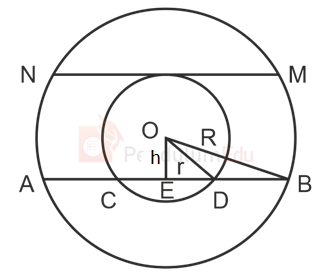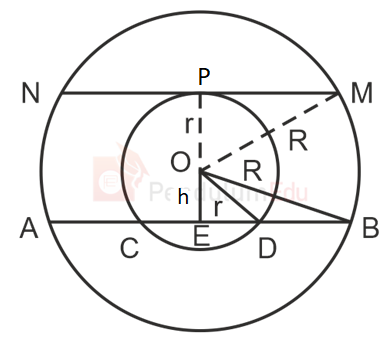Question of The Day06-09-2021

In the given figure, AB and MN are two chords such that, AB = 58 cm and CD = 42 cm. What is the value of MN?Correct Answer : c ) 40 cm

Explanation :

According to the question

Let, ‘O’ be the center of the concentric circles, ‘r’ and ‘R’ be the radius of smaller and larger circle

Respectively,

OE is drawn perpendicular to AB and let OE be ‘h’We know that,

If a perpendicular is drawn from the center to any chord of the circle, then it bisects the chord and vice versa.

⇒ED=$$CD \over 2$$=$$42 \over 2$$

⇒ ED = 21 cm

Similarly,

⇒EB=$$AB \over 2$$=$$58 \over 2$$

⇒ EB = 29 cm

In ∆OED (using Pythagoras theorem)

⇒ OD2 = OE2 + ED2

Substituting the values

⇒ r2 = h2 + 212………..(1)

In ∆OEB (using Pythagoras theorem)

⇒ OB2 = OE2 + EB2

⇒ R2 = h2 + 292……….(2)In △OPM (using Pythagoras theorem)

OM2 = OP2 + PM2

Substituting the values

⇒ R2 = r2 + PM2

⇒ PM2 = R2 – r2

Substituting the values for eq(1) and eq (2)

⇒ PM2 = (h2 + 292) – (h2 + 212)

⇒ PM2 = h2 + 292 – h2 – 212

⇒ PM2 = 292 – 212

⇒ PM2 = 400

⇒PM=$$\sqrt {400}$$

⇒ PM = 20 cm

⇒ NM = 2PM

⇒ NM = 2 * 20

⇒ NM = 40 cm

Hence, (c) is the correct answer.0